# Addition and Subtraction in Scientific Notation

Author: Sophia Tutorial
##### Description:

Add or subtract numbers in scientific notation.

(more)

Sophia’s self-paced online courses are a great way to save time and money as you earn credits eligible for transfer to many different colleges and universities.*

No credit card required

37 Sophia partners guarantee credit transfer.

299 Institutions have accepted or given pre-approval for credit transfer.

* The American Council on Education's College Credit Recommendation Service (ACE Credit®) has evaluated and recommended college credit for 33 of Sophia’s online courses. Many different colleges and universities consider ACE CREDIT recommendations in determining the applicability to their course and degree programs.

Tutorial
what's covered
1. Addition and Subtracting in Scientific Notation
2. Adding and Subtracting with Common Powers of 10
3. Adding and Subtracting with Unlike Powers of 10

# 1. Addition and Subtracting in Scientific Notation

Scientific notation can be used to express very large and very small numbers by multiplying a decimal number by a power of 10. By very large number, we mean a number containing several digits to the left of the decimal, such as 23,000,000 (23 million). By small number, we mean a number with zero in the ones place, with several decimal digits behind it, such as 0.000023 (23 millionths).

Generally, numbers written in scientific notation can be expressed as a • 10^n, where a is a decimal number, and 10^n represents a power of ten.

In proper scientific notation, there are a couple of restrictions to what “a” is allowed to be. “a” can only contain one non-zero digit to the left of the decimal. Here are some examples of numbers that might look like they are in scientific notation, but they violate this rule for what “a” can be: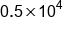A zero to the left of the decimal is not allowed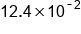More than 1 digit to the left of the decimal is not allowed

In cases where there is a zero or more than one digit to the left of the decimal, we can move the decimal and adjust the exponent. Let’s change our previous examples into proper scientific notation:A zero to the left of the decimal is not allowed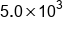Decimal moved right 1 place, exponent decreased by 1More than one digit to the left of the decimal is not allowed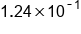Decimal moved left 1 place, exponent increased by 1

big idea
When moving the decimal in scientific notation, movement to the left increases the power of 10, and movement to the right decreases the power of 10.
hint
Be careful when increasing and decreasing negative powers of 10. For example, decreasing -3 by 1 makes the number more negative: -4. Similarly, increasing -3 by 1 makes the number less negative: -2.

# 2. Adding and Subtracting with Common Powers of 10

How does this help us add and subtract numbers in scientific notation? We can easily add and subtract numbers in scientific notation if they are expressed using the same power of 10. This is illustrated in the following example: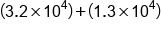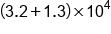Add the decimal numbers together, keep the power of 10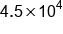Our Solution

We can confirm this is a valid process by showing the addition in standard notation: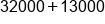Writing in standard notation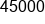Adding the numbersOur solution in scientific notation

hint
Sometimes when you add the decimal numbers, you will get a sum that does not fit the rules for proper scientific notation. Remember to always check that a single non-zero digit is to the left of the decimal. If necessary, move the decimal and adjust the exponent.
big idea
When the powers of 10 are the same, we can add and subtract numbers in scientific notation following the rule a x 10n + b x 10n = (a+b) x 10n

# 3. Adding and Subtracting with Unlike Powers of 10

What if we wish to add or subtract numbers in scientific notation that have unlike powers of 10? We can use our handy trick of moving the decimal and adjusting the exponent to express the numbers with a common power of 10. This is illustrated in the following examples: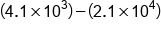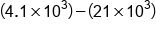Rewrite to have a common power of 10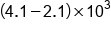Group the decimal numbers, keep power of 10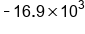Subtract 21 from 4.1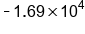Our Solution

Note that it does not matter which number you choose to rewrite. We would get the same result if we chose to rewrite the other number, although we might give ourselves more work to do. Let’s go through the same example, but in a different way: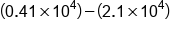Rewrite one number to have a common power of 10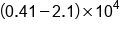Subtract decimal numbers, keep power of 10Our Solution

​As you can see in the second example, we needed to make a final adjustment to our solution, because -16.9 x 10^3 is not in proper scientific notation. No matter which number you decide to rewrite, always be sure to make any necessary movements to the decimal, and increase or decrease your exponent accordingly.

hint
This tip is worth repeating, especially when adding or subtracting numbers in scientific notation. During the process, you will probably work with numbers that are not written in proper scientific notation. This is okay, because it is required to create a common power of 10. Just be sure that you make final adjustments to your expression, so that the decimal number contains only a single non-zero digit to the left of the decimal. Remember, decimal movement to the left increases the power of 10, and decimal movement to the right decreases the power of 10.
summary
When you're adding and subtracting numbers that are written in scientific notation with common powers of 10, meaning the powers of 10 are the same, you can just add or subtract your decimal numbers, and keep the same power of 10. When adding or subtracting numbers in scientific notation with unlike powers of 10, we can rewrite one of the numbers by moving the decimal and increase or decreasing the power of 10. Move the decimal either to the left if you want to increase the exponent or move it to the right if you want to decrease the exponent. This creates two numbers with a common power of 10, which can be added or subtracted by combining the decimal numbers and keeping the common power of 10.

Source: Adapted from "Beginning and Intermediate Algebra" by Tyler Wallace, an open source textbook available at: http://wallace.ccfaculty.org/book/book.html

Rating